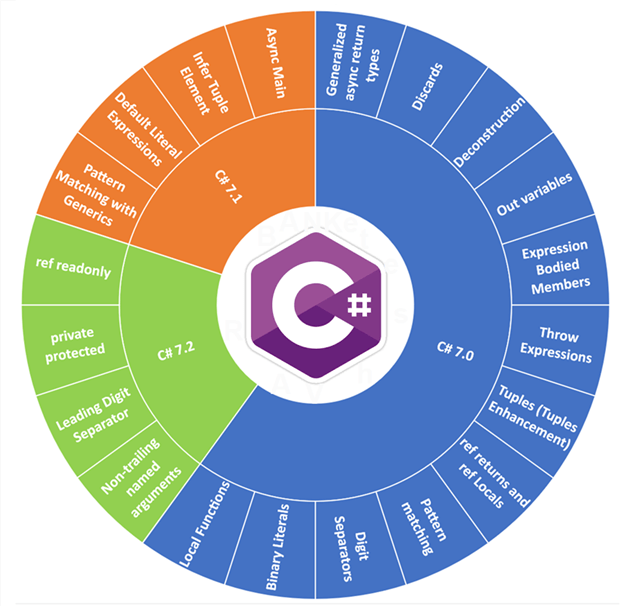### 零度 C#7.0 中的语言新特性### 1、Out 变量

```static void Main(string[] args)
{
int value; //需要预先定义
if (int.TryParse("123", out value))
{
Console.WriteLine(value);
}
}```

```static void Main(string[] args)
{
if (int.TryParse("123", out int value))
{
Console.WriteLine(value);
}
}```

### 2、元组特性

`install-package System.ValueTuple`

```public static (string v1, string v2, string v3) GetValues()
{
return ("零度", "编程", "官网");
}
```

```public static (string, string, string) GetValues()
{
return ("零度", "编程", "官网");
}```

```(string a, string b, string c) = GetValues();
Console.WriteLine(a + b + c);```

```var result = GetValues();
Console.WriteLine(result.v1 + result.v2 + result.v3);```

### 3、解析方法

```(string first, string middle, string last) = GetValues();
Console.WriteLine(first + middle + last);```

`(var first, var middle, var last) = GetValues(); `

`var (first, middle, last) = GetValues();`

`public void Deconstruct(out T1 x1, ..., out Tn xn) { ... }`

```public class Book
{
public int BookID { get; set; }
public double BookPrice { get; set; }
public void Deconstruct(out int id, out double price) { id = BookID; price = BookPrice; }
}```

```Book book = new Book { BookID = 123, BookPrice = 88.88 };
(int id, double price) = book;
Console.WriteLine(price);```

### 4、模式匹配

```static void Main(string[] args)
{
object objectValue = 123;
if (objectValue is int)
{
int intValue = (int)objectValue;
int sum = intValue + 321;
Console.WriteLine(sum);
}
}```

```static void Main(string[] args)
{
object objectValue = 123;
if (objectValue is int intValue)
{
int sum = intValue + 321;
Console.WriteLine(sum);
}
}```

```static dynamic AddValue(object value)
{
dynamic result=null;
switch (value)
{
case int b:
result = b++;
break;
case string c:
result = c + "零度";
break;
}
return result;
}```

### 5、局部函数

```static void Main(string[] args)
{
{
return value1 + value2;
}
Console.WriteLine(result);
}```

### 6、数值文字

```static void Main(string[] args)
{    var intValue = 123_456_000_000; //表示123456000000    var hexValue = 0xAB_CD_EF; //0x表示十六进制
var binValue = 0b1010_1011_1100_1101_1110_1111; //0b表示二进制
}```

### 7、方法主体使用表达式

```public class Person
{
public void PrintName() => Console.WriteLine(firstName);
private string firstName = string.Empty;
public Person(string name) => firstName=name; //构造函数
~Person() => firstName=string.Empty; //析构函数
public string FirstName
{
get => firstName; //GET访问器
set => firstName = value; //SET访问器
}
}```

### 8、异常表达式

```public void WriteString(string parameter)
{
if (parameter == null)
{
throw new ArgumentNullException();
}
Console.WriteLine(parameter);
}```

```public void WriteString2(string parameter)
{
parameter = parameter?? throw new ArgumentNullException();
Console.WriteLine(parameter);
}```

### 9、将 ref 用于变量和返回值

```static void Main(string[] args)
{
int x = 3;
ref int y = ref x;
y = 2;
Console.WriteLine(x);
}```

`static ref int GetValue(int[] array, int index) => ref array[index];`

```int[] array = { 1, 2, 3, 4, 5 };
ref int x = ref GetValue(array, 2);```